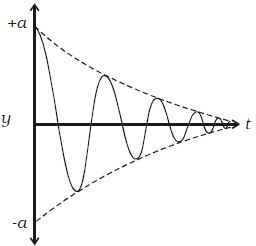# Types of Oscillations

Oscillation is defined as the process of repeating variations of any quantity or measure about its equilibrium value in time. The most common examples for oscillation are the tides in the sea and the movement of a simple pendulum in a clock.

Oscillation can also be defined as a periodic variation of a matter between two values or about its central value. Another example of oscillation is the movement of spring. The vibration of strings in guitar and other string instruments are also examples of oscillations.

There are three main types of oscillations.

(i) Free oscillations When a body vibrates with its own natural frequency, it is said to execute free oscillations. The frequency of oscillations depends on the inertial factor and spring factor, which is given by,

n = 1/2π √(k/m)

Examples: (i) Vibrations of a tuning fork

(ii) Vibrations in a stretched string

(iii) Oscillations of a simple pendulum

(iv) Airblown gently across the mouth of a bottle.

(ii) Damped oscillations: Most of the oscillations in the air or in any medium are damped. When an oscillation occurs, some kind of damping force may arise due to friction or air resistance offered by the medium. An oscillation remains undamped when a restoring force equal to the restraining for is induced and hence the system oscillates with the same energy. So, a part of the energy is dissipated in overcoming the resistive force. Consequently, the amplitude of oscillation decreases with time and finally becomes zero. Such oscillations are called damped oscillations (Figure). When the restoring force is not applied the oscillation suddenly stops. And when the restoring force applied is less than​ the restraining force, damping is introduced.Examples:

(i) The oscillations of a pendulum

(ii) Electromagnetic damping in galvanometer (oscillations of a coil in galvanometer)

(iii) Electromagnetic oscillations in the tank circuit

(iii) Maintained oscillations: The amplitude of an oscillating system can be made constant by feeding some energy to the system. If energy is fed to the system to compensate for the energy it has lost, the amplitude will be a constant. Such oscillations are called maintained oscillations (Figure).Example: A swing to which energy is fed continuously to maintain the amplitude of oscillation.

(iv) Forced oscillations

When a vibrating body is maintained in the state of vibration by a periodic force of frequency (n) other than its natural frequency of the body, the vibrations are called forced vibrations. The external force is driver and body is driven.

Examples :

(i) Sound boards of stringed instruments execute forced vibration,

(ii) Press the stem of vibrating tuning fork, against tabla. The tabla suffers forced vibration.

(v) Resonance

In the case of forced vibration, if the frequency difference is small, the amplitude will be large. Ultimately when the two frequencies are same, amplitude becomes maximum. This is a special case of forced vibration.

If the frequency of the external periodic force is equal to the natural frequency of oscillation of the system, then the amplitude of oscillation will be large and this is known as resonance.